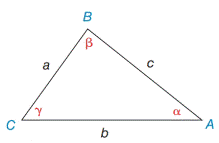Chapter 11.4, Problem 2EElementary Geometry For College St...

7th Edition
Alexander + 2 others
ISBN: 9781337614085

Solutions

Chapter
SectionElementary Geometry For College St...

7th Edition
Alexander + 2 others
ISBN: 9781337614085
Textbook Problem

In Exercises 1 and 2, use the given information to find an expression for the area of Δ A B C . Give the answer in a form such as A = 1 2 ( 3 ) ( 4 )  sin 32 ∘ . See the figure for Exercises 1 to 8.a) b = 7.3 ,   c = 8.6 ,  and  α = 38 ∘ b) a = 5.3 ,   c = 8.4 ,   α = 36 ∘  and  γ = 87 ∘Exercises 1-8

To determine

a)

To find:

The area of the ABC in the form of A=12(a)(b) sin α

If a=7.3, b=8.6, and α=38

Explanation

1) Approach:

The area of an acute triangle equals one-half the product of the lengths of two sides and the sine of the included angle.

The area of the triangle ABC is given by,

A=12absinα

2) Calculation:

Given,

In triangle ABC,

a=7.3b=8.6 andα=38

The area of the triangle ABC A=12absinα

A=12(7

To determine

To find:

The area of the ABC in the form of A=12(a)(b) sin γ

If a=5.3, b=8.4, α=36 and γ=87

Still sussing out bartleby?

Check out a sample textbook solution.

See a sample solution

The Solution to Your Study Problems

Bartleby provides explanations to thousands of textbook problems written by our experts, many with advanced degrees!

Get Started

8. Find all horizontal and vertical asymptotes of the function .

Mathematical Applications for the Management, Life, and Social Sciences

f(x)1xx24

Applied Calculus for the Managerial, Life, and Social Sciences: A Brief Approach

The average value of f(x) = 3x2 + 1 on the interval [2, 4] is: a) 29 b) 66 c) 58 d) 36

Study Guide for Stewart's Single Variable Calculus: Early Transcendentals, 8th

For

Study Guide for Stewart's Multivariable Calculus, 8th